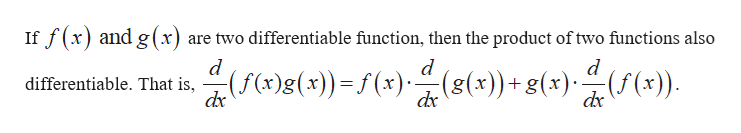# Find the derivative of the function.f(x) = x6 ln(x)

Question
1 views

Find the derivative of the function.

f(x) = x6 ln(x)
check_circle

Step 1help_outlineImage TranscriptioncloseIf f(x) and g(x) are two differentiable function, then the product of two functions also d d (f()g(x)(x) (g(x))+ g(x) (f(x). differentiable. That is, dx dx fullscreen

### Want to see the full answer?

See Solution

#### Want to see this answer and more?

Solutions are written by subject experts who are available 24/7. Questions are typically answered within 1 hour.*

See Solution
*Response times may vary by subject and question.
Tagged in

### Derivative